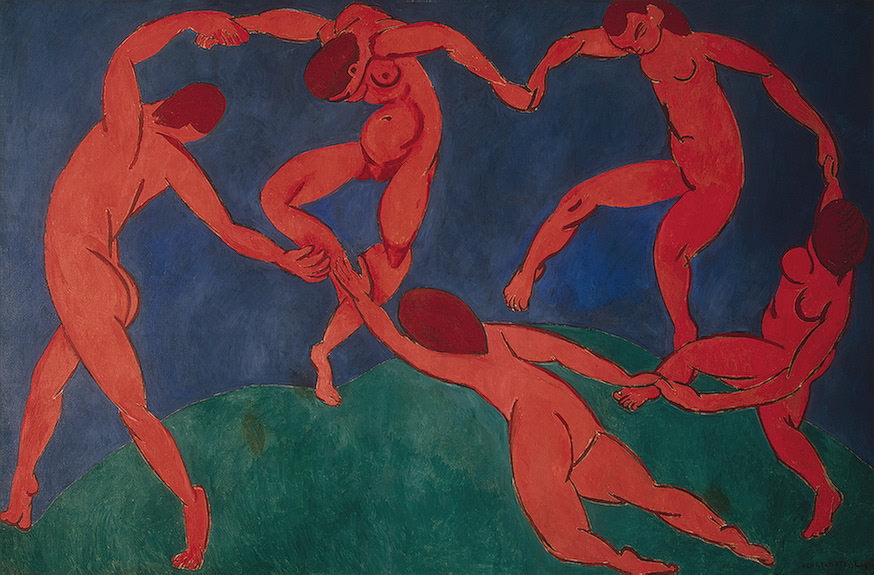Several of my past papers concern Lombardi drawing, which I and my coauthors named after conspiracy-theory artist Mark Lombardi. In this style of drawing, edges are drawn as circular arcs, and must meet at equal angles around every vertex. Not every graph has such a drawing, but many symmetrical graphs do (example below: the smallest zero-symmetric graph with only two edge orbits).All 2-degenerate graphs do as well; these are the graphs that can be reduced to nothing by removing vertices of degree at most two. And all 3-regular planar graphs have planar drawings in this style; I drew the one below to illustrate Grinberg’s theorem, a necessary condition for Hamiltonicity of planar graphs.So anyway, my newest arXiv preprint is “Bipartite and series-parallel graphs without planar Lombardi drawings” (arXiv:1906.04401, to appear at CCCG). It is about some families of planar graphs that have Lombardi drawings (because they are 2-degenerate) but do not have planar Lombardi drawings. They include planar bipartite graphs like the one below (but with more edges and vertices):and embedded series-parallel graphs like the one below (again, with more edges and vertices):The key structures that makes both of these graphs hard to draw in Lombardi style are their yellow-blue quadrilateral faces. The red parts of the graph are just filler to make these faces have the right angles. The yellow-blue quadrilaterals all share the same two yellow vertices, and I like to think of them as forming a ring dancing hand-to-hand around these two yellow vertices like Matisse’s dancers.When I wrote about quadrilaterals with circular-arc edges meeting at equal angles at each vertex last December, it was these rings of quadrilaterals I was thinking about. As I wrote in my previous post, each of these quadrilaterals has a circumscribing circle. It’s not hard to make one arc polygon with four equal angles, as sharp as you like. But when the angles get sharp and two of these polygons share two opposite vertices and are packed at a tight angle next to each other (as all the yellow-blue quadrilateral faces in these graphs do) each of the two adjacent quadrilaterals must have a deep pocket into which its neighbor reaches to touch its circumcircle, and this forces them to become quite distorted looking.If we think of these two quadrilaterals as dancers, with their heads and toes at the shared points and with their hands free, then both of them have their right hands (from the perspective of the viewer) held high and their left hands held low. Extending the same picture to a complete ring of dancers, each dancer in the ring must be holding their right hand higher than their neighbor to the left. But this obviously can’t extend all the way around the ring, because when you came back to the dancer you started with their hand should be the same height as it was when you started.

This is all very metaphorical but fortunately this intuition can be turned into a mathematical proof that the drawing doesn’t exist. The correct tools for measuring the heights of the quadrilateral vertices turn out to be Möbius transformations and bipolar coordinates, a system for assigning coordinates to points in the plane by the angle they make with two fixed points (the two yellow shared vertices of all the quadrilateral faces of our graph) and the ratio of their distances to the fixed points.Möbius transformations preserve the circular-arc shape of our quadrilateral sides, and the angles at which they meet. When we restrict them to the transformations that leave the two poles of the bipolar coordinate system fixed, they act very nicely on the two coordinates, essentially adding fixed amounts to each coordinate. We can use them to transform our quadrilaterals into a more canonical shape and prove that the radius-ratio coordinate increases from one quadrilateral to the next around our ring of quadrilaterals, getting the same contradiction described above and proving that no drawing exists.

The most obvious questions in the same direction that this paper leaves unsolved are: what about series-parallel graphs where you do not have a fixed planar embedding for the graph? And what about outerplanar graphs (either with the outerplanar embedding or without fixing an embedding)? We neither have a method for finding planar Lombardi drawings of all graphs of these types, nor a proof that these drawings do not exist.